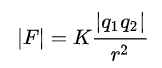# Is Electrostatic Force Conservative: 3 Important Concepts

An electrostatic force which is also called as coulombic force is conservative in nature. Let us see how is electrostatic force conservative in detail below.

The electrostatic force, also known as the coulombic force, is conservative in nature, which means that the total amount of work done to move charge q from one place to another is independent of the path taken.

## How is electrostatic force conservative?

Before understanding how is electrostatic force conservative, first, let us understand the meaning of conservative force.

• Conservative force: A force is said to be conservative when the total effort required to transport a particle between two positions is unaffected by the particle’s path.
• So, let us return to the actual discussion of whether electrostatic force is conservative.
The explanation is that the Electrostatic force is conservative since it satisfies the principle mentioned above and does not violate it. Let us demonstrate this with an example.

## Conservation of electrostatic force:

Let us consider the system of two charges out of which we are taking a unit positive charge ‘Q’ from position A to position B in a straight line and then from position b to position A by any other path, as shown in the figure below. Then the work done in taking charge from one place to another over a closed path should be equal to zero. If it so happens then, that means that electrostatic force is conservative.

Now from above figure we can write that, if we take charge Q from A to B,

WAB = the work done in taking charge Q from position A to position B

WAB  = Q (VA – VB) …… (1)

Similarly, if we take charge Q from B to A,

WBA  = the work done in taking charge Q from position B to position A

WBA = Q (VB – VA) …… (2)

(Since the potential difference is the work done per unit charge)

So, the total or net work done over a closed path can be calculated as,

W = WAB+ WBA

Therefore by adding equation 1 and equation 2 we get,

W = Q (VA – VB) + Q (VB – VA)

∴ W = Q [ VA – VB + VB – VA ]

∴ W = Q 

∴ W = 0

The total work done is zero because the effort required to move charge Q from A to B is not dependent on the path, and hence the electrostatic force is said to be conservative.

Note: We already learned that electrostatic attraction between two point charges always works along the line that connects them and that it is also an action-reaction pair. This is why electrostatic force is referred to as a Central Force. And, once again, it is a well-established truth that any central force is by nature conservative.

## Q. What is electrostatic force or coulombic force ?

Ans: To compute the force that occurs between two electrically charged particles that are stationary in space.

“The law states that the magnitude of the electrostatic force of attraction or repulsion between two point charges is directly proportional to the product of the magnitudes of charges and inversely proportional to the square of the distance between them.”Where  K (Coulomb’s constant) ≈ 8.988×10⁹ N⋅m²⋅C⁻²,

q1 and q2 = magnitudes of the charges

and r = distance between the two charges.

When two charges are connected by a straight line, the force is directed in a straight path between them. The exerted force is repulsive when the charges are identical, and the force is attractive in the case of opposite charges.

## Q. What is the meaning of an electric field?

Ans: The field which is encountered by other charged particles.

An electric field is a field or vicinity where a charged particle feels some force exerted on it.

Electric fields can be produced by electric charges or by time-varying magnetic fields, depending on their source.

The electric field is given by,

E = F/q

Where, E = Electric field, F = Coulomb force and q = unit test charge

## Q. What are the different examples of conservative forces?

Ans: Following are the examples of conservative forces

## Q. What are the examples of electrostatic force?

Ans: We can observe electrostatic force in our day to day life objects.

1. When we rub the comb on oily hair on the head and then if that comb is placed near the tiny pieces of paper then, it’s observed that the tiny pieces get attracted to the comb. This happens because of the electrostatic force generated in between these objects.
2. If two balloons rubbed on each other, then they seem to get attracted to each other.
3. Lighting in the sky.

## Q. What is the meaning of force and field?

Ans: The force and the field are two different things.

Force: A force is something that can set the body in motion after the application of it.

Field: A field is a mathematical concept or entity that refers to a space in which each point has a value.

Prajakta Gharat

I am Prajakta Gharat. I have completed Post Graduation in physics in 2020. Currently I am working as a Subject Matter Expert in Physics for Lambdageeks. I try to explain Physics subject easily understandable in simple way.